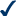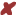Global searching is not enabled.

## WFM Glossary

#### Workforce Management Terminologies, definitions, formulas and Erlang calculators!

Browse the glossary using this index

A | B | C | D | E | F | G | H | I | J | K | L | M | N | O | P | Q | R | S | T | U | V | W | X | Y | Z | ALL

### O

#### Occupancy

The time that an agent is handling calls (talk time plus wrap-up) divided by the total time that the agent is available for handling calls. It can be calculated by; net workload / gross workload or net workload / net workforce. Not to be confused with utilization or productivity.

#### OR

Operations Research, the science that uses mathematical models to improve business operations. Also known as Management Science, therefore sometimes called OR/MS. See also www.informs.org.

#### Order of Operations

When we speak about operations mathematically, it means things like add, subtract, multiply, divide, squaring, etc. Remember: If it isn't a number it is probably an operation.

When you see something like ...

7 + (6 × 52 + 3)

... what part should you calculate first?

Start at the left and go to the right?

Or go from right to left?

Warning: Calculate them in the wrong order, and you will get a wrong answer !

So, long ago people agreed to follow rules when doing calculations, and they are:
Do things in Parentheses First. Example:6 × (5 + 3) = 6 × 8 = 486 × (5 + 3) = 30 + 3 = 33 (wrong)
Exponents (Powers, Roots) before Multiply, Divide, Add or Subtract. Example:5 × 22 = 5 × 4 = 205 × 22 = 102 = 100 (wrong)
Multiply or Divide before you Add or Subtract. Example:2 + 5 × 3 = 2 + 15 = 172 + 5 × 3 = 7 × 3 = 21 (wrong)
Otherwise just go left to right. Example:30 ÷ 5 × 3 = 6 × 3 = 1830 ÷ 5 × 3 = 30 ÷ 15 = 2 (wrong)
How Do I Remember It All ... ? PEMDAS !
 P Parentheses first E Exponents (ie Powers and Square Roots, etc.) MD Multiplication and Division (left-to-right) AS Addition and Subtraction (left-to-right)

source: mathisfun.com